# Scottish Cancer Patient Experience Survey 2015/16

Results from the 2015/16 Scottish Cancer Patient Experience Survey. The survey covers the full care journey that a cancer patient experiences, from thinking that something might be wrong with them to the support they received after their acute-care treatm

### Annex B: Significance testing

a. Comparison of results between most and least deprived SIMD quintiles

For each question where responses could be classed as positive or negative (see Annex C) significance testing was conducted to see if the percentage of those living in the least deprived SIMD quintile was significantly different from those living in the most deprived quintile.

The test statistic can be calculated as follows: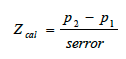To illustrate this, let the:

• percentage positive score for the most and least deprived SIMD quintiles be denoted by p 1 and p 2 respectively.
• number of valid responses from most and least deprived SIMD quintiles be denoted by n 1 and n 2 respectively.

The standard error can be calculated as: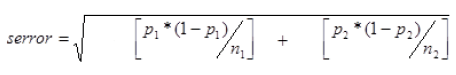For a result in the most deprived quintile to not be statistically significantly different from the least deprived quintile, then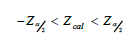For the purpose of this testing, α was 0.05

b. Tumour group scores

For each question where responses could be classed as positive or negative (see Annex C) a Pearson chi-square test was used to detect any statistically significant differences in the distribution of positive responses between tumour groups (α = 0.05). This excluded patients where the tumour group was unknown, or their tumour type was included in the 'other' category.

Where statistically significant differences were found, significance testing was conducted to see if each tumour group was significantly different from the national (or 'all-cancer') average. As per the SIMD analysis, the test statistic can be calculated as follows: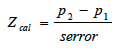To illustrate this, let the:

• percentage positive score for all respondents and the tumour group in question be denoted by p 1 and p 2 respectively.
• number of valid responses from all respondents and the tumour group in question be denoted by n 1 and n 2 respectively.

The standard error can be calculated as: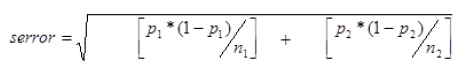A result for a particular tumour group was not statistically significantly different from the national (all-cancer) figure whereFor the purpose of this testing, α was 0.05